Type
Solution Manual
Book Title
Managerial Economics 8th Edition
ISBN 13
978-1118808948

### 978-1118808948 Chapter 16 Solution Manual

June 14, 2019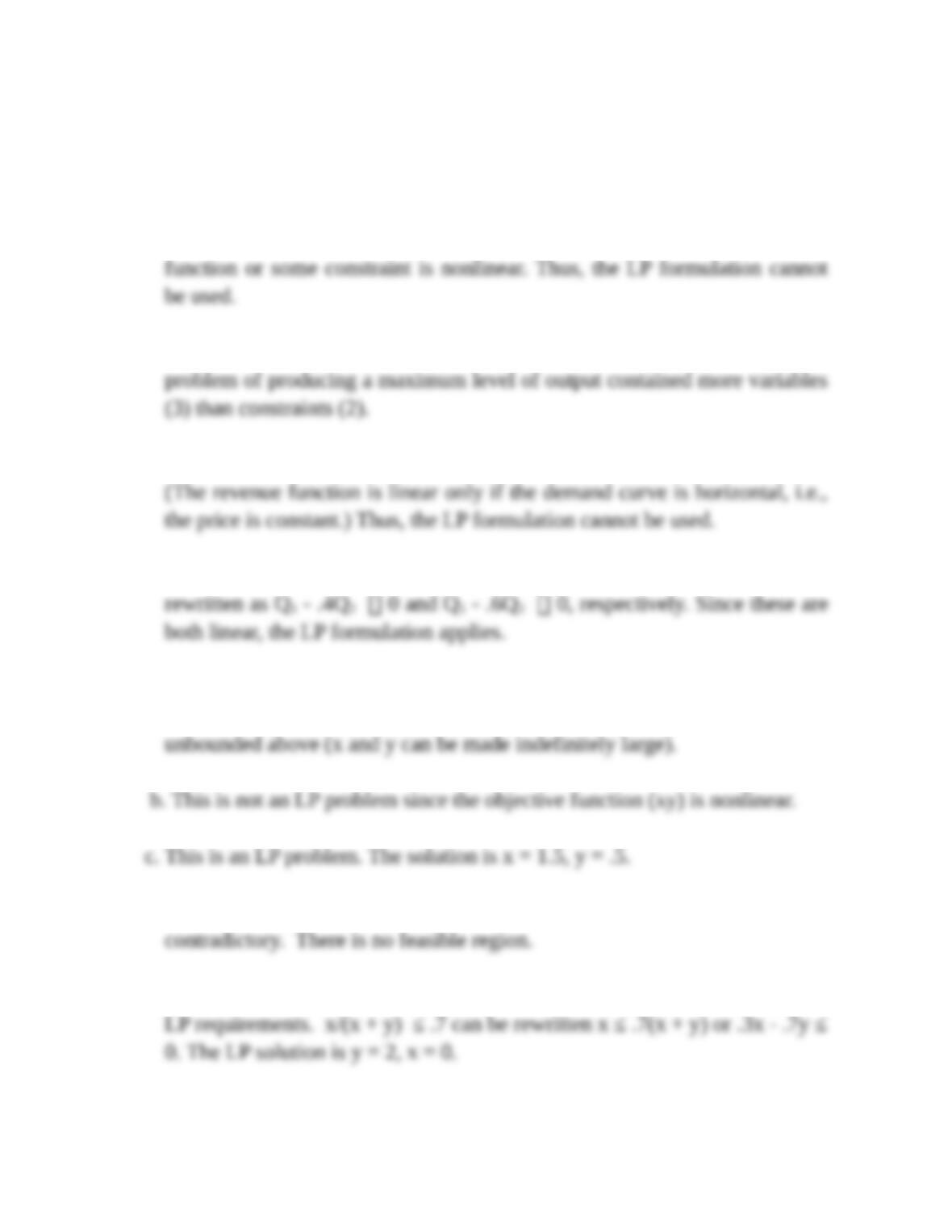1. a. Increasing or decreasing returns to scale implies that either the objective
b. The LP method can handle any number of decision variables. The earlier
c. A downward-sloping demand curve implies a nonlinear revenue function.
d. Here, the constraints are Q1/Q2 .4 and Q1/Q2 .6. These can be
2. a. This maximization problem cannot be solved since the feasible region is
d. This maximization problem has no solution since the inequalities are
e. The second inequality (though it looks strange) can be rearranged to fit the
John Wiley & Sons
17-8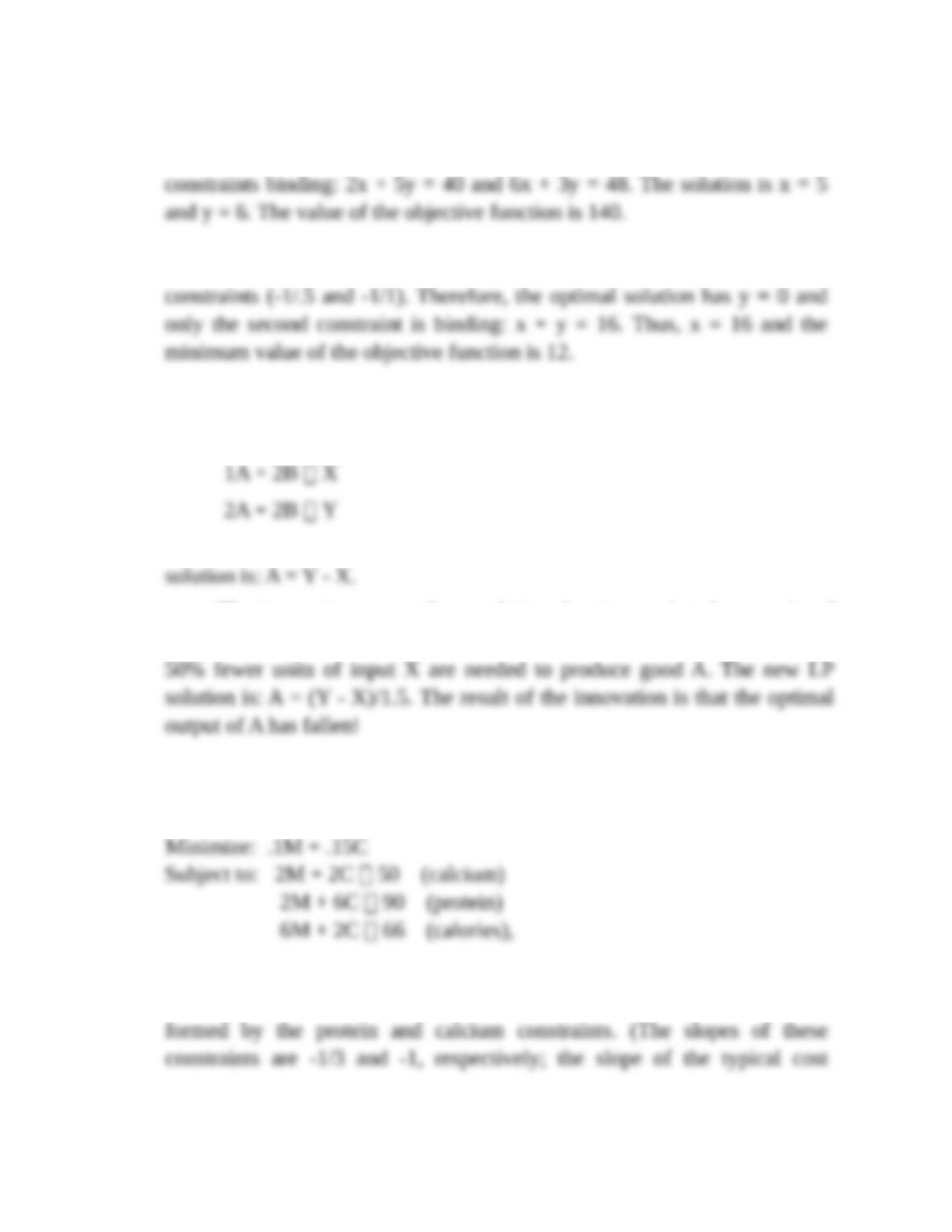3. a. The slope of the objective function (-10/15) lies between the slopes of the
two constraints (-2/5 and -6/3). Therefore, the optimal solution has both
b. The slope of the objective function (-.75) lies outside the slopes of the two
4. Let the initial resource constraints be:
and assume that both are binding in the optimal solution. Note that the LP
The innovation means that producing A units requires fewer units of
good X. For instance, the new constraint might be: 5A + 2B X, so that
5. a. The formulation is
where M and C are the nonnegative quantities of milk and cereal. A graph
shows that the lowest contour touches the feasible region at the corner
John Wiley & Sons
17-8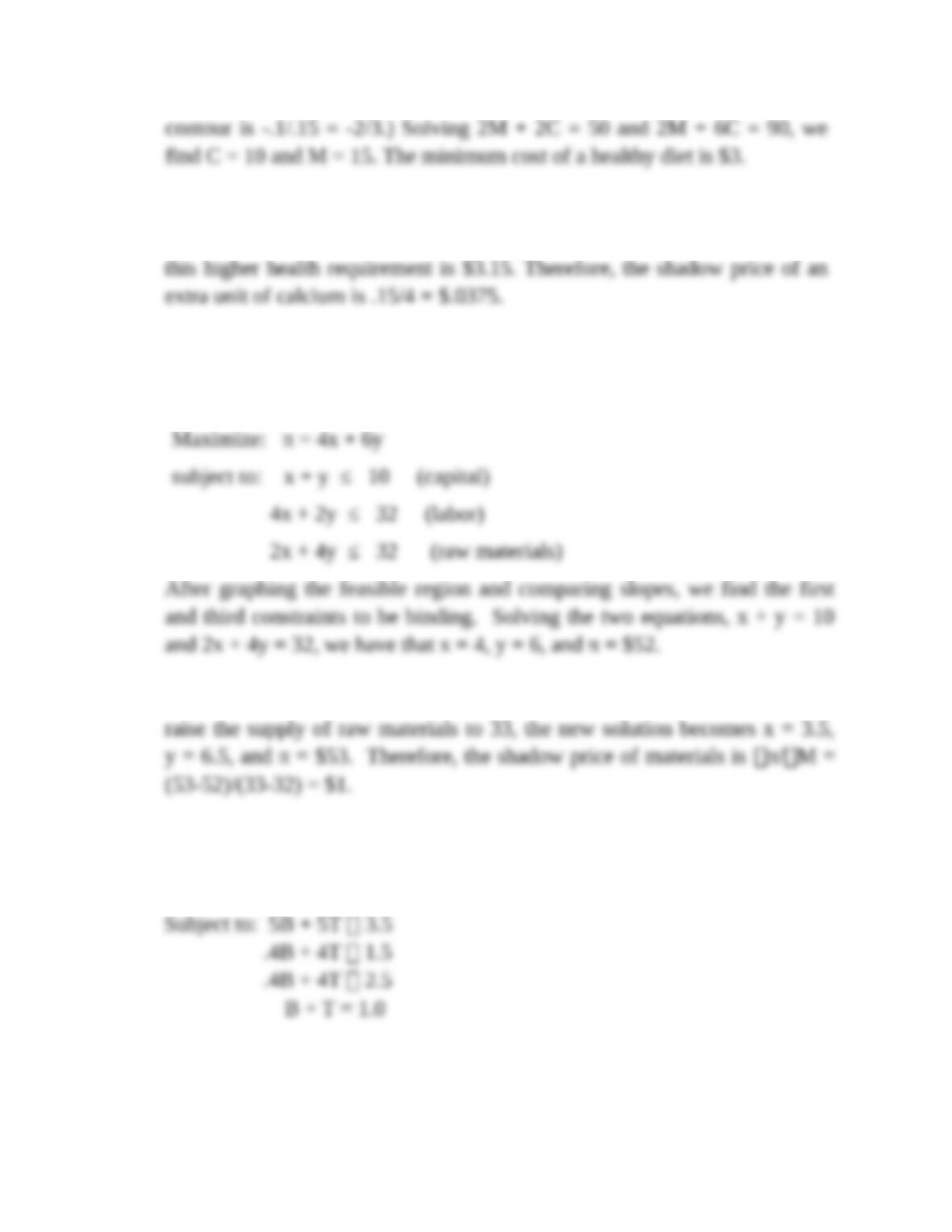b. If we increase the calcium requirement by a small amount (say, by 4 units
to 54), the new solution becomes C = 9 and M = 18. The cost of meeting
6. a. Let x and y be the numbers of tires produced via processes 1 and 2
respectively. The LP formulation is:
b. Since the labor constraint is not binding, labor's shadow price is zero. If we
7. a. The formulation is
Maximize: 4B + 6T
Since bonds have better returns, the investor would like to make T as large
John Wiley & Sons
17-8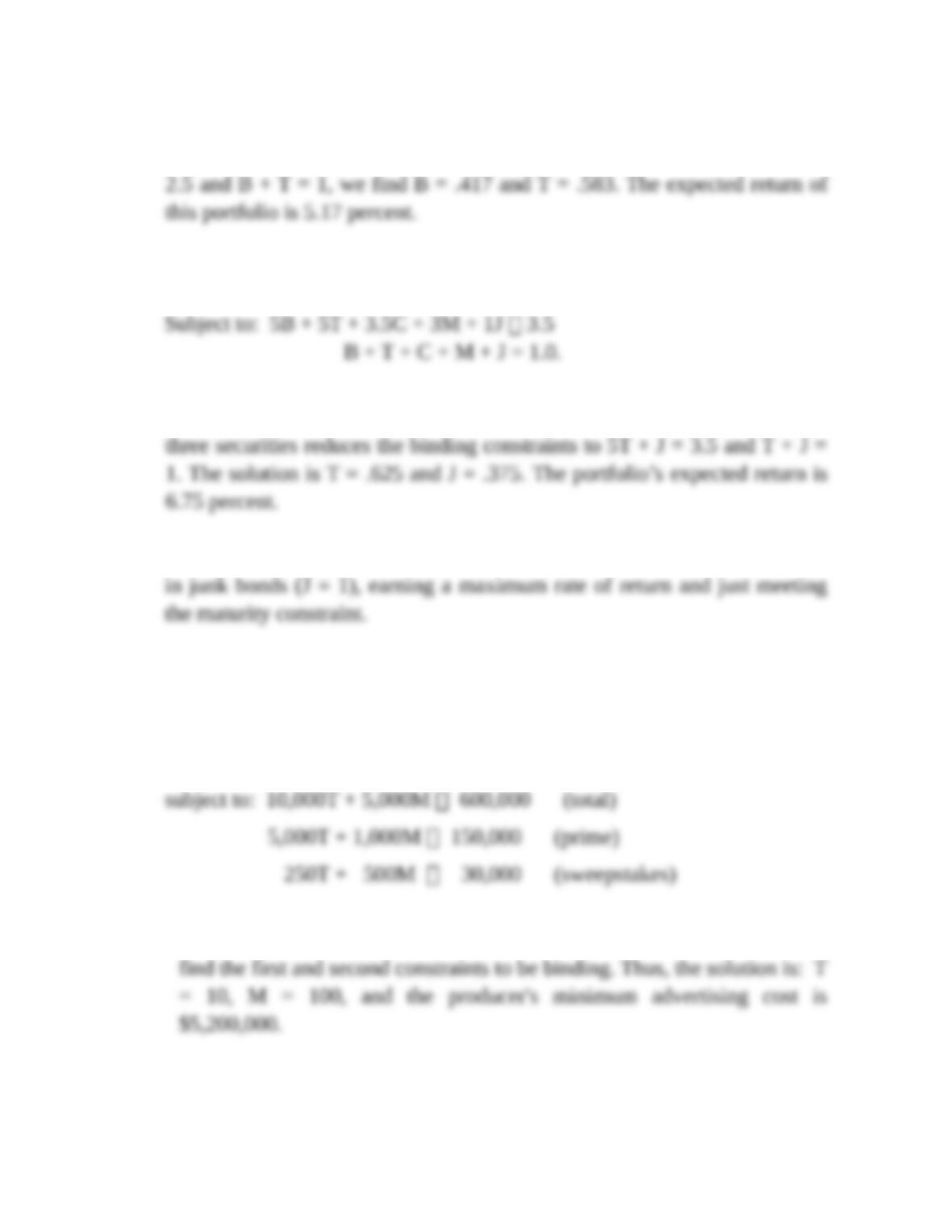as possible. Clearly, the first two constraints never are binding. However,
the last two constraints do bind the proportion of bonds. Solving .4B + 4T =
b. The formulation is
Maximize: 4B + 6T + 4.4C + 5.6M + 8J
Notice that Treasury bonds dominate (are more profitable and safer) than
Treasury bills, corporate bonds, and municipal bonds. Eliminating these
c. If risk is not an issue, the manager should invest 100 percent of the portfolio
8. Let T and M be the numbers of TV and magazine ads respectively. The LP
formulation is:
Minimize: Cost = 120,000T + 40,000M
Note that this is a minimization problem involving “greater to or equal”
constraints. After graphing the feasible region and comparing slopes, we
9. a. Let x1 and x2 denote the levels of the two processes. At a unit level, process
1 produces 2 units of H and 1 unit of P for a total contribution of (\$2)(2) +
John Wiley & Sons
17-8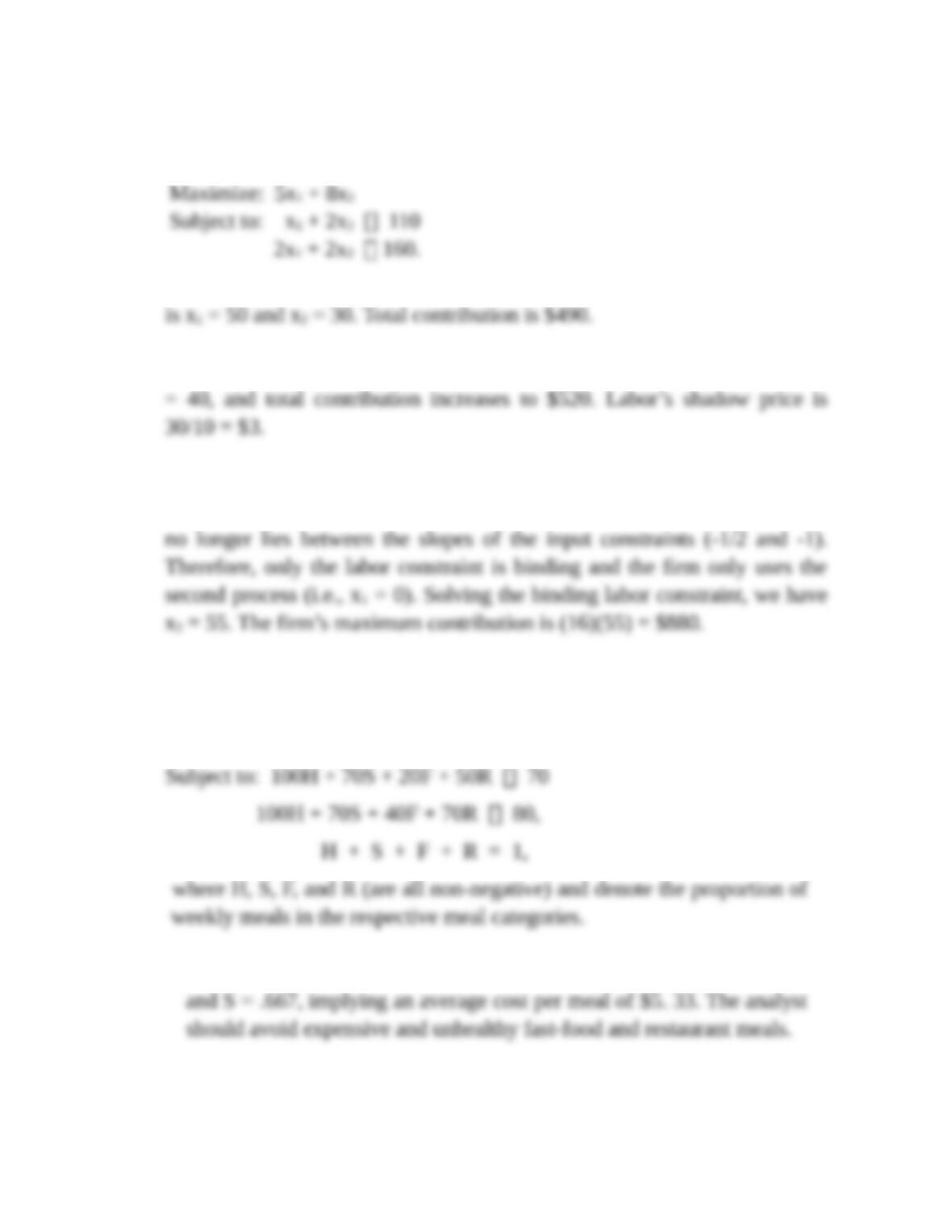(\$1)(1) = \$5. The contribution of process 2 is (\$2)(2) + (\$1)(4) = \$8 at the
unit level. Thus, the LP formulation is
In the graphic solution, both constraints are binding. The optimal solution
b. Let the supply of labor increase to 120. The new solution is x1 = 40 and x2
c. If the contribution of plywood rises to \$3, the new objective function
becomes maximize 7x1 + 16x2. The slope of the objective function (-7/16)
10. a. The LP formulation is
Minimize: 6H + 5S + 8F + 20R
b. The minimum cost weekly meal plan calls for the proportions, H = .333
c. Now the LP formulation is
John Wiley & Sons
17-8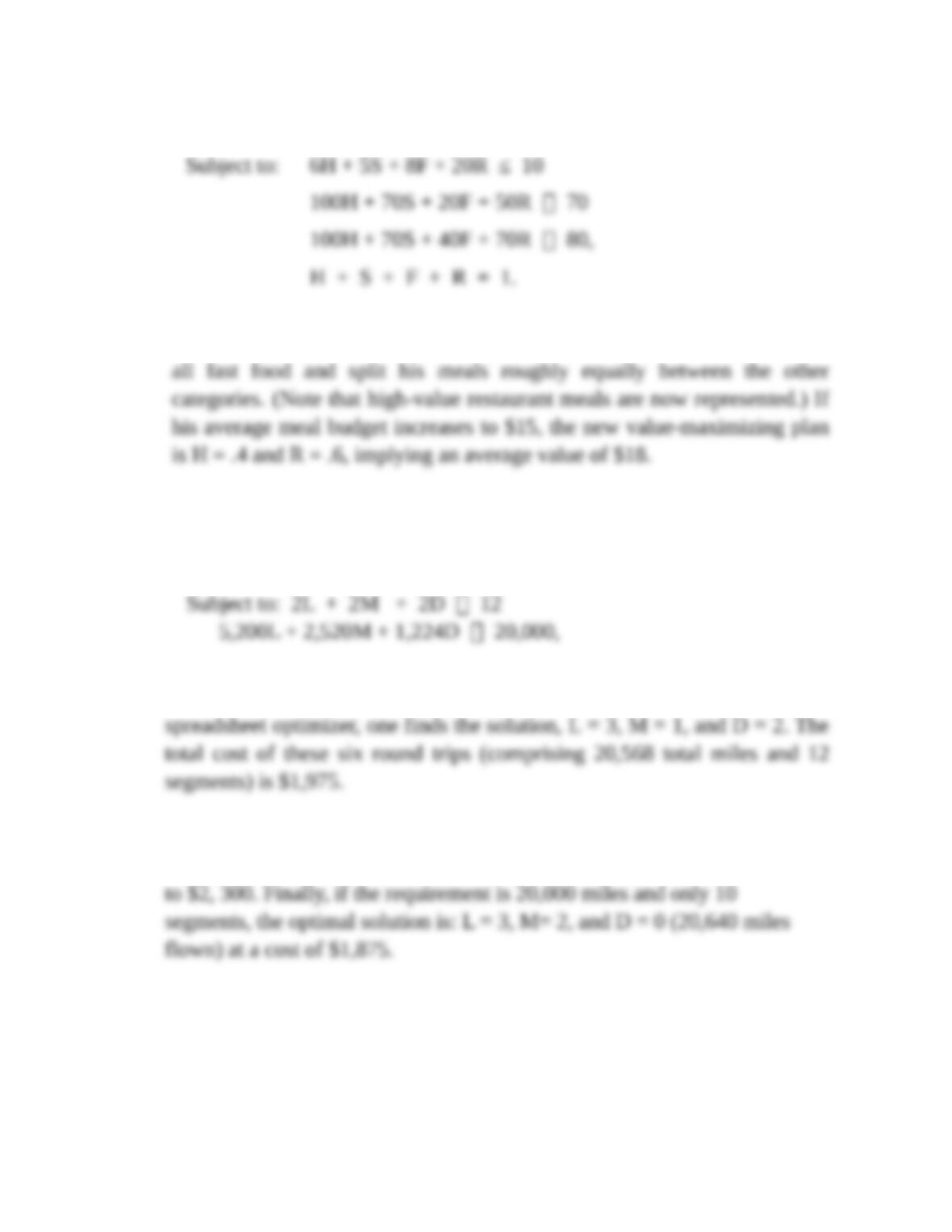Maximize: 3H + 8S + 12F + 28R
The maximum value meal plan (average value is \$12.56) calls for the
proportions, H = .33, S = .36, F = 0, and R = .31. The analyst should avoid
11. a. The LP formulation is
Minimize: 425L + 300M + 200D
where L, M, and D (are all non-negative integers) and denote the number of
roundtrips to Los Angeles, Miami, and Durham, respectively. Using a
b. If the challenge is to fly 25,000 miles, the best solution is: L = 4, M= 2,
and D = 0. The total cost of these trips (covering 25,840 miles) increases
.
Discussion Question
John Wiley & Sons
17-8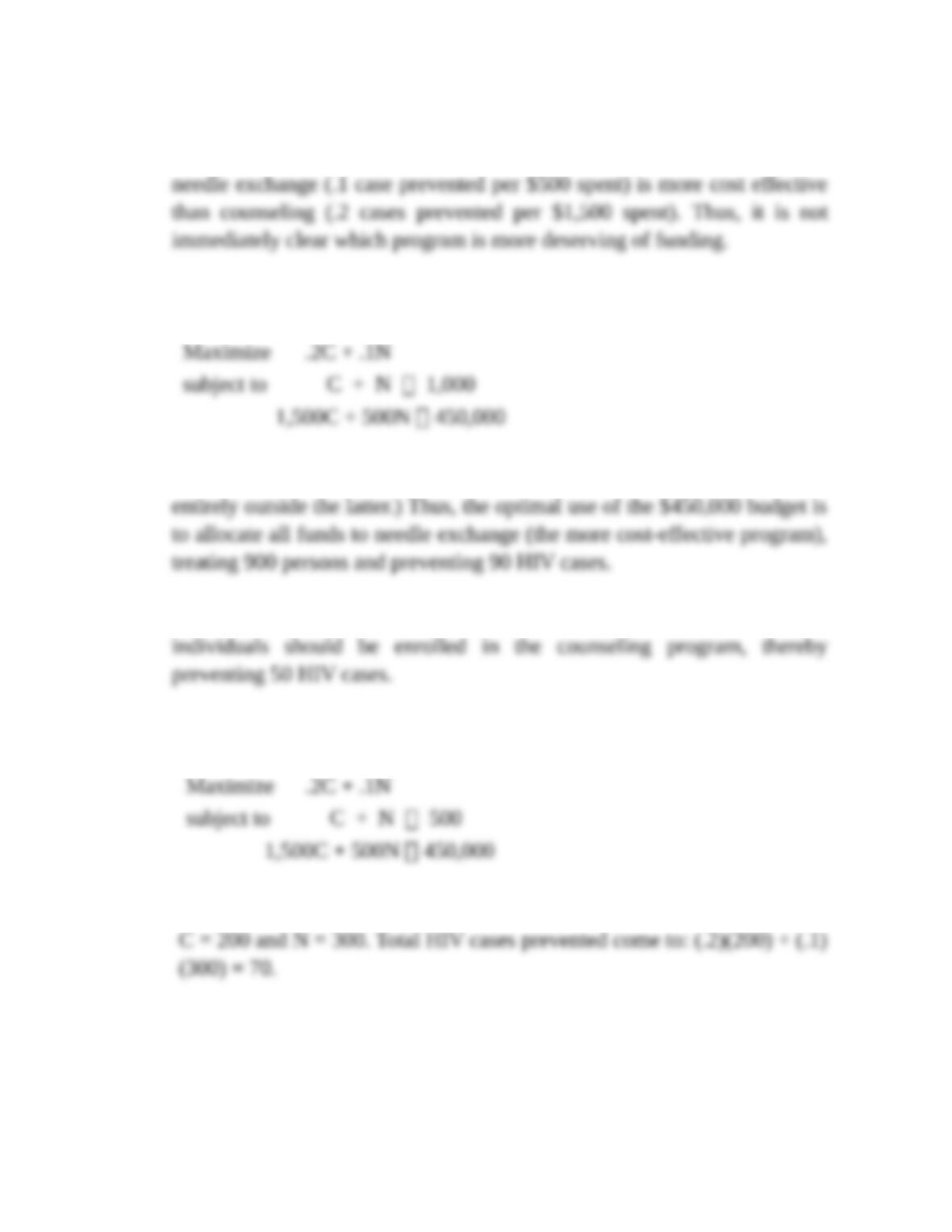a. Counseling (preventing .2 new HIV cases per enrollee) is more effective
than needle exchange (preventing .1 new case per enrollee). However,
b. The LP formulation is
Graphing the two constraints shows that the former is never binding (it lies
c. Now the sole binding constraint is: C + N 250. All 250 at-risk
d. Now, the LP formulation is
The optimal corner has both constraints binding, implying the allocation,
S1. a. The LP formulation is:
John Wiley & Sons
17-8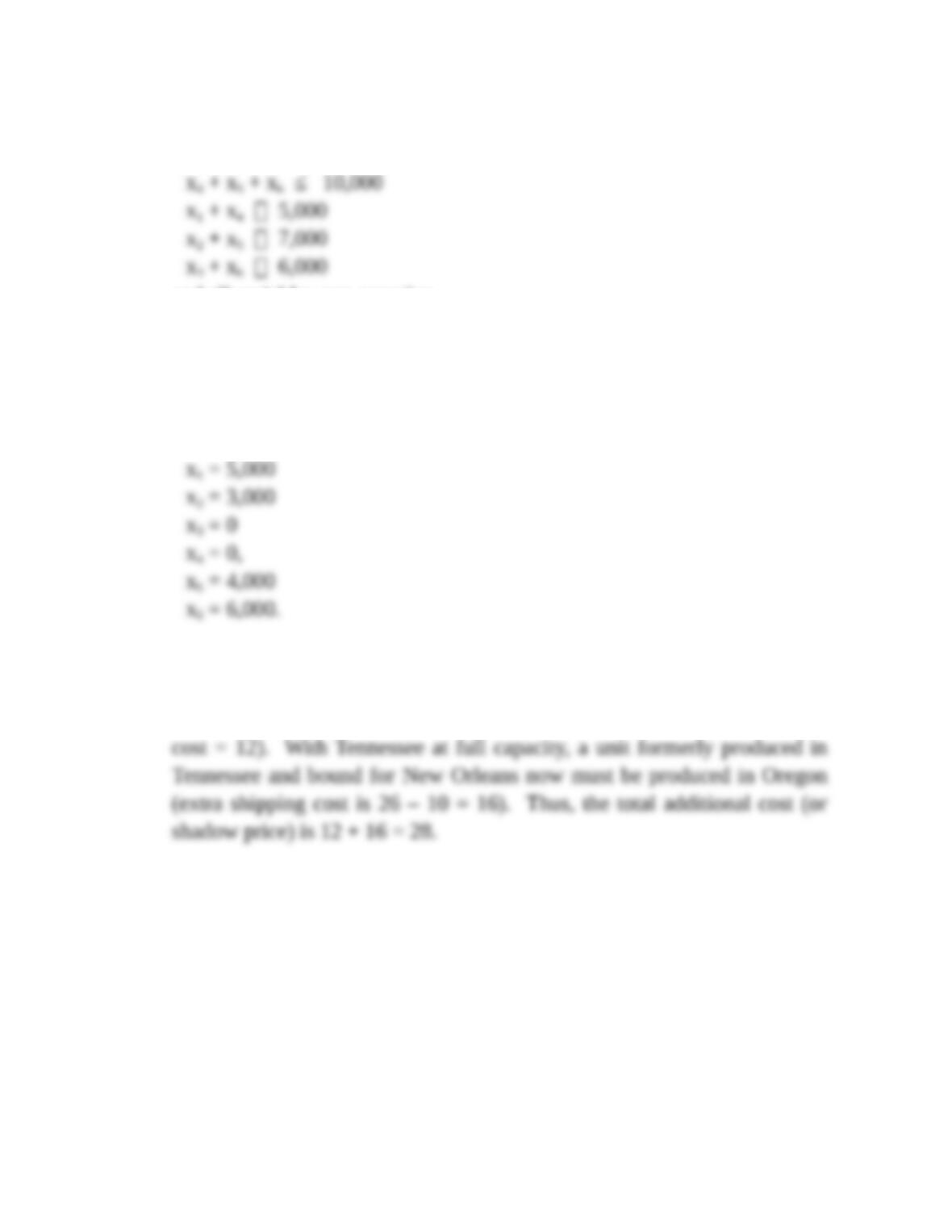minimize Cost = 14x1 + 26x2 + 30x3 + 24x4 + 10x5 + 12x6
subject to: x1 + x2 + x3 ≤ 9,000
and all variables non-negative.
b. Using the spreadsheet optimizer, we minimize the total transport cost in
cell H6 by changing cells C13, D13, E13, C14, D14, and E14 subject to
cells C21, D21, E21, I13, and I15 all being greater or equal to zero. The
optimal solution is:
c. Using the optimizer, we find the shadow price associated with shipments to
New York to be 28. The explanation for the shadow price is as follows.
The extra unit shipped to New York is produced in Tennessee (shipping
John Wiley & Sons
17-8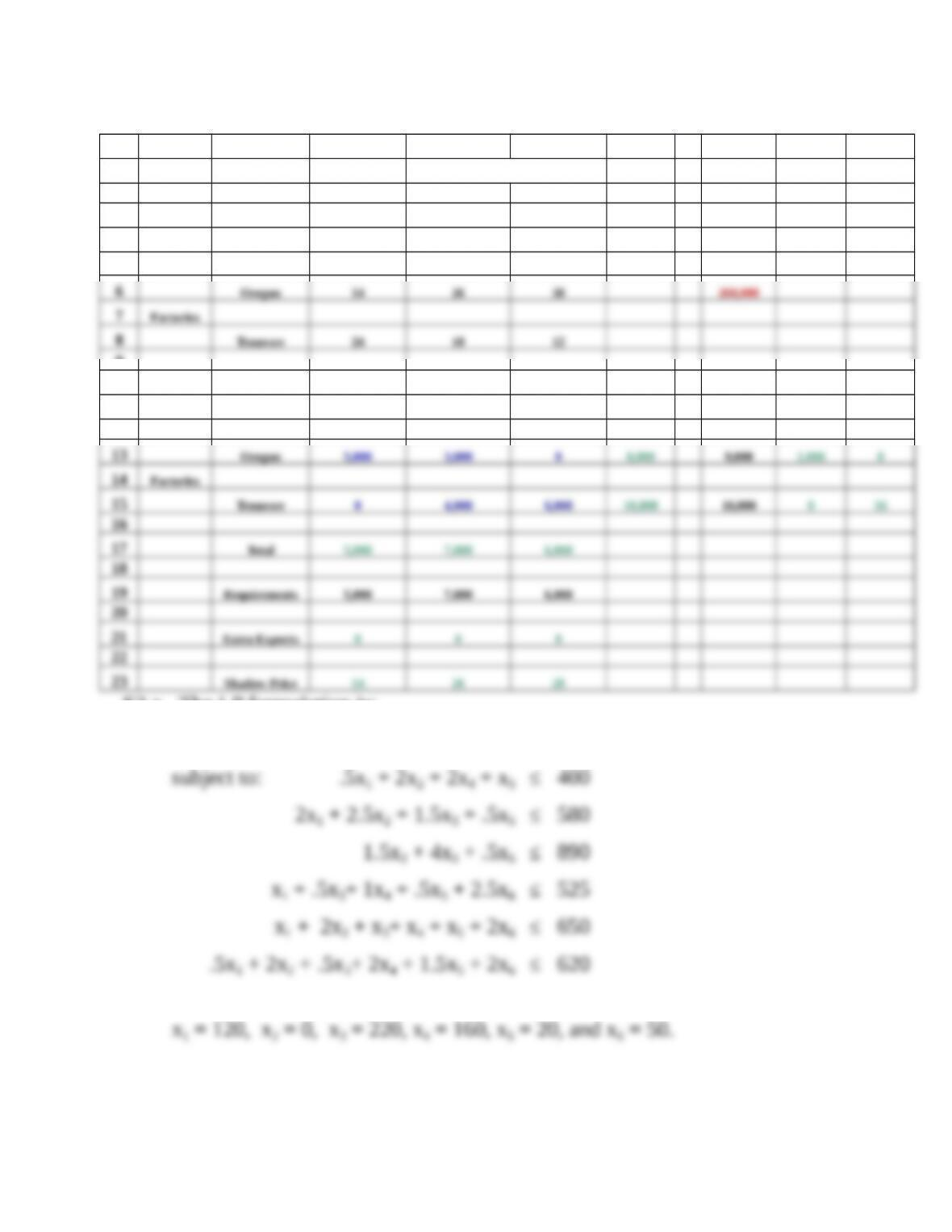S2 a. The LP formulation is:
maximize = 60x1 + 70x2 + 48x3 + 52x4 + 48x5 + 60x6
b. From the spreadsheet optimizer, the optimal solution is:
John Wiley & Sons
17-8
A B C D E F G H I J
1A SHIPPING PROBLEM
2
3 Transport Ports Minimum
4Costs Los Angeles New Orleans New York Cost
5
9
10 Units Shipped Ports Factory Unused Shadow
11 Los Angeles New Orleans New York Total Capacity Capacity Price
12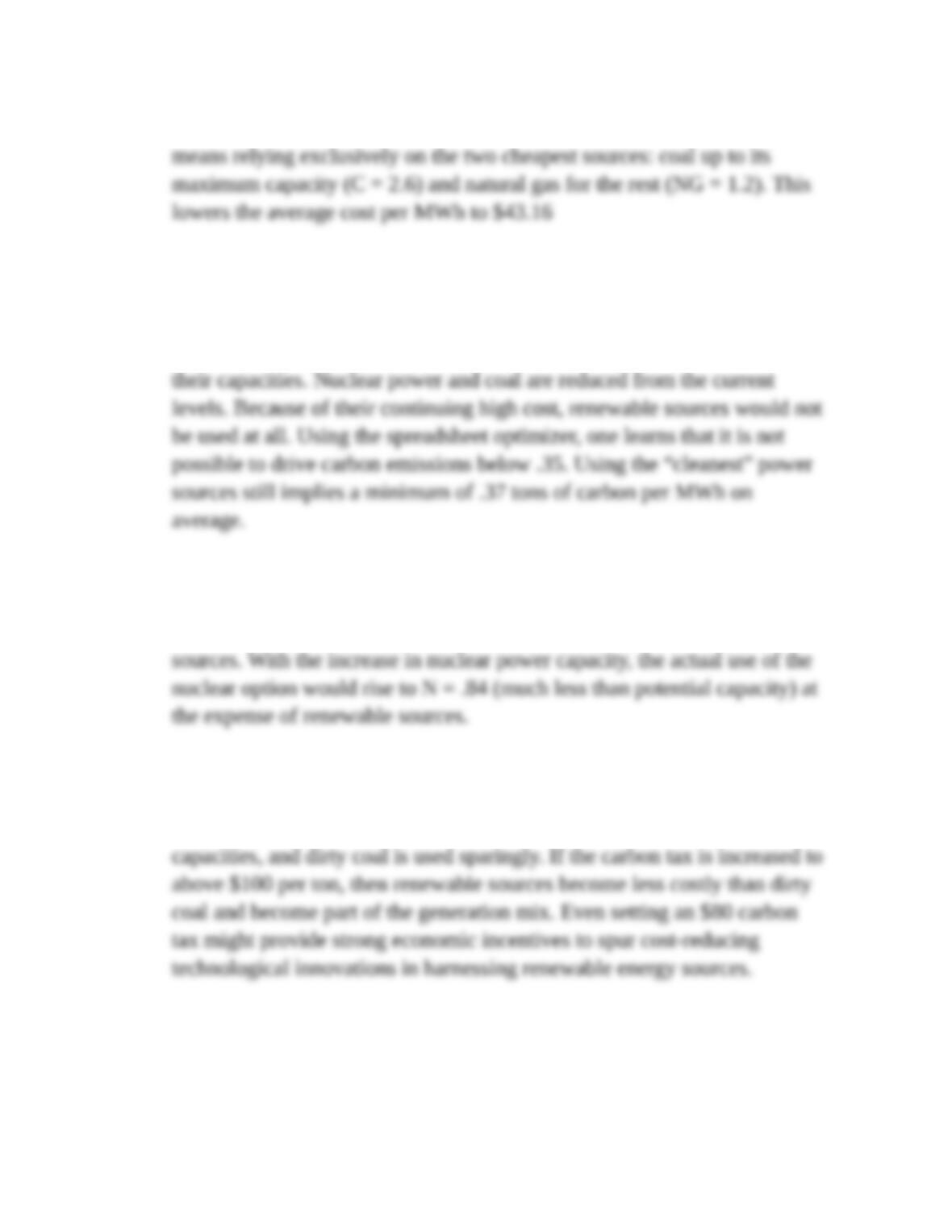S3. a. Annual generation of 3.8 billion MWh of electricity at minimum cost
b. Adding the constraint that carbon emissions must average below .5 tons
per MWh implies the solution: C = .43, CC = .5, NG = 1.9, N = .53, H = .
44, and R = 0. Clean coal, natural gas (which are both relatively cheap and
clean) and hydroelectric power (clean but expensive) are all used up to
c. Using the spreadsheet optimizer while honoring both constraints, one finds
the solution: C = .08, CC = .5, NG = 1.9, N = .75, H = .44, and R = .13.
Dirty coal is nearly eliminated from the mix in favor of the “cleaner”
d. With an \$80 carbon tax in place, the costs of the various power sources
(per MWh) inclusive of the tax become: \$128, \$104, \$77, \$115, \$100, and
\$150 respectively. The four cheapest sources are used up to their
S4. a. Absent the possibility of trade, the US sets its outputs (cells C8 and D8)
to maximize its total value (cell E17) subject to spending no more than its
John Wiley & Sons
17-8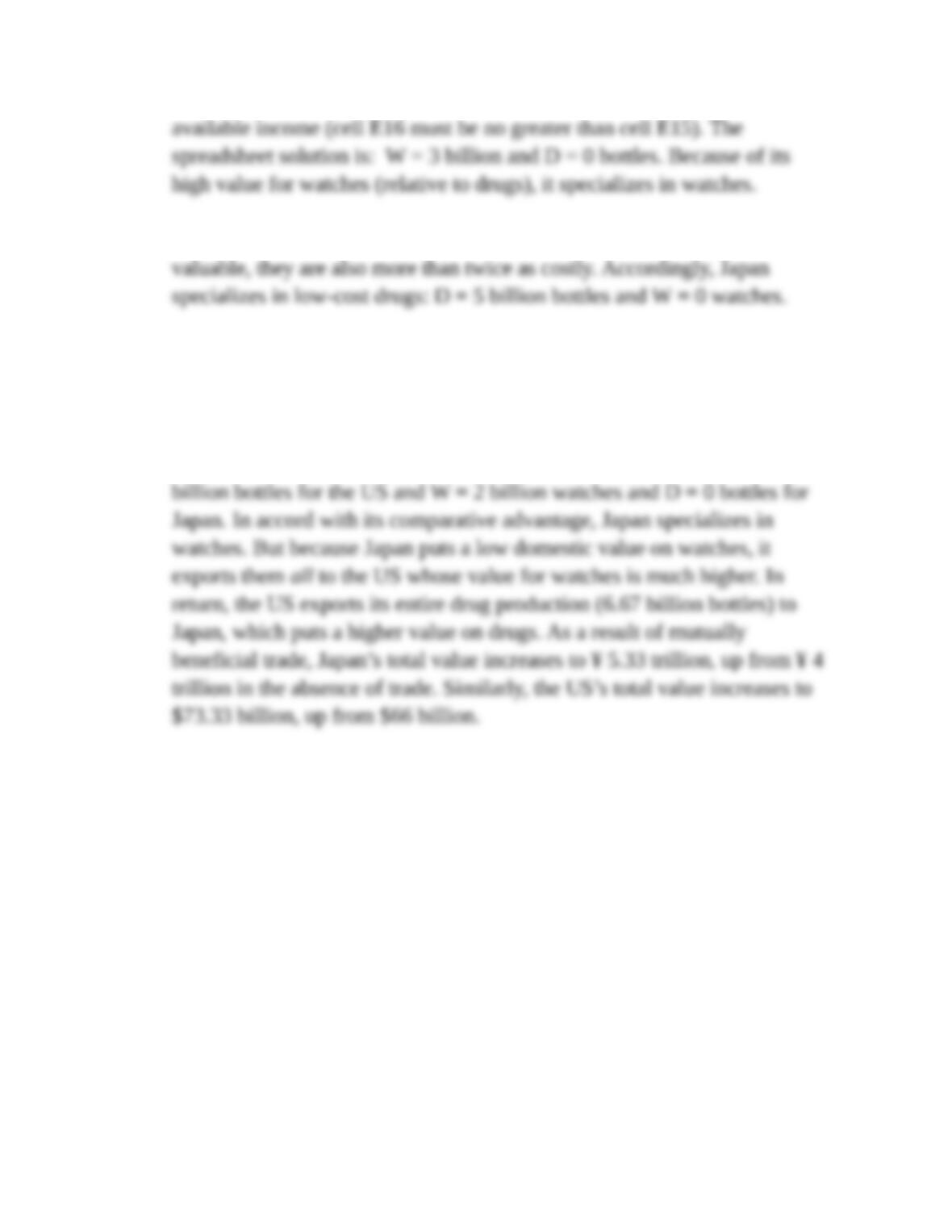b. In Japan, the situation is exactly the reverse. Though watches are twice as
c. Now, the LP solution treats both countries’ outputs and trade in the goods
as decision variables with the objective of maximizing the value of total
consumption in the countries subject to balanced trade in each. (Balanced
trade imposes the constraints, cell C13 = -cell D13.) Using the spreadsheet
optimizer, one finds the solution: W = 1.33 billion watches and D = 6.67
John Wiley & Sons
17-8